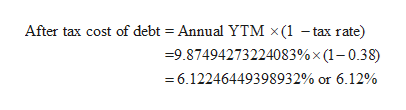# Simple Foods has a zero coupon bond issue outstanding that matures at nine years. The bonds are selling at 42% of par value. What is the company's after tax cost of debt if the tax rate is 38%? (Use semi annual compounding)

Question
71 views

Simple Foods has a zero coupon bond issue outstanding that matures at nine years. The bonds are selling at 42% of par value. What is the company's after tax cost of debt if the tax rate is 38%? (Use semi annual compounding)

check_circle

Step 1

The company has a zero coupon bond that matures in 9 years with semiannual compounding.

Par Value (FV) = \$1,000

Price (P) = 42% * \$1000 = \$420

Semiannual YTM ( r ) = to be determined

Number of periods (n) = 9*2 = 18

Yield to maturity can be calculated as below:

Step 2

Annual YTM is semiannual YTM multiplied by 2.

Step 3

After tax cost of debt is c...help_outlineImage TranscriptioncloseAfter tax cost of debt = Annual YTM x(1 -tax rate) =9.87494273224083% x (1-0.38) 6.12246449398932% or 6.12% fullscreen

### Want to see the full answer?

See Solution

#### Want to see this answer and more?

Solutions are written by subject experts who are available 24/7. Questions are typically answered within 1 hour.*

See Solution
*Response times may vary by subject and question.
Tagged in